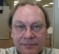## inverse quantizationStarted by 4 years ago6 replieslatest reply 4 years ago1387 views

I understand the process of quantization but I am unable to understand inverse quantization process.

Can someone please provide some background into this?

Thanks,

[ - ]I confess complete ignorance of the term "inverse quantization".  I googled and found two two references whose contexts related to DCT of images. Is that what you are asking? Or are you referring to the difference between ADC ("quantization") and it inverse DAC processes? Until you clarify the meaning of your question, I expect it will go unanswered. The problem statement can be 50% of the solution, if done correctly.

[ - ]Artmez,

Yes, I am referring to IQ in the context of DCT and image processing.

[ - ]Quantization maps continuous values to members from a discrete set.  For encoding, each element in that set is represented by an integer.  These integers are what are encoded and transmitted by codecs.  IQ (inverse quantization) maps these integers back to their corresponding values from discrete set used by the quantizer.

Example:

Consider a simple quantizer that contains the following set of values {-1.1, 0.2, 0.9, 2.3}.  Say you want to encode 1.2.  You would likely choose the closest value in this set as the quantized value, i.e. 0.9.  It is the third value in the list, so we encode it using the integer 2 (assuming the first entry is assigned to 0).  The decoder receives the 2, looks it up in the quantizer set/table and sees that it represents 0.9.  The process of converting the 2 to 0.9 is inverse quantization.

[ - ]I'm assuming you're referring to inverse quantization process as in audio, video or image CODECs.

if that is the case, if you know quantization then you can guess inverse quantization.

for example, in JPEG encoding, the DCT coeff are computed from the pixel matrix. the coeff computed are zig-zag scanned, dc removed and entropy coded. since entropy coding used in JPEG is huffman, it's required to convert the coeff into integers within a specified *limit*. as you may know, in this case, quantization is the process of converting the DCT coeff into integer value within limit. JPEG std is providing a quantization matrix for this purpose. similar scheme is used in several other encoding such as MP3, AAC, MPEG2 video, etc.

now, the inverse quantization is the opposite of this. ie, for example, for JPEG, at the decoder, before feeding to the IDCT, one need to convert the quantized numbers back into the original range so that they are numerically *correct* so that IDCT can use it in the IDCT formula.

[ - ]Sounds like you may be referring to "reconstruction" of signal after it is being "quantized".

If that is the question, then you would need to learn about sampling theory and conditions about reconstructing a quantized signal.

Signals are quantized for number of reasons

1. Limited memory space to store them

2. Transmitting digital information has more immunity to noise and distortion, more tolerable to smaller SNR and/or SINR for successful reconstructing the signal

3. Digital information can be secured by coding

Best regards,

Shahram Shafie

[ - ]